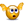# Logarithmic Trend Line

## Excel Help for Logarithmic Trend Line in Analyzing Corporate Financial DataInstead of adding the linear trend line to the scatter plot series, you can add a logarithmic trend line.  When displaying the equation and r-squared, you will get the Log(t) (where "x" replaces t) and the p-reg is represented by "y" in the equation as the dependent variable of the regression equation. Excel Business Forums Administrator Posted by Excel Helper on 26 Sep 2010